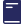Over 1000 Titles for students & teachers

# Excel Year 11 Mathematics Advanced Study Guide

Bestseller
\$34.95

#### About This Book

This brand new full-colour format has even more features than before to help students succeed in Year 11.

Chapters:

• Topic 1: Functions
• Chapter 1: Working with functions
• Topic 2: Trigonometric functions
• Chapter 2: Measure of angles, trigonometric functions and identities
• Topic 3: Calculus
• Chapter 3: Introduction to differentiation
• Topic 4: Exponential and logarithmic functions
• Chapter 4: Logarithms and exponentials
• Topic 5: Statistical analysis
• Chapter 5: Probability and discrete probability distributions
• Sample Year 11 examination papers
• Answers
• Index
• #### Product Details

SKU:
9781741256796
YEAR:
Year 11
BRAND:
Excel
SUB BRAND:
Excel Year 11
SUBJECT:
Mathematics
TYPE:
Study Guides